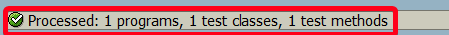# How to create unit tests for ABAP global class in SE24?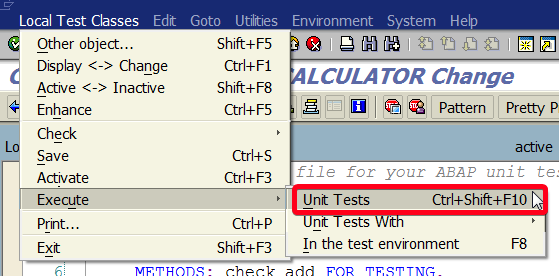In this tutorial, you will learn how to create a unit test for global ABAP class in transaction SE24.

Step 1. To create a unit test for global ABAP class go to transaction SE24 (Class Builder. Put se24in the command field and hit Enter: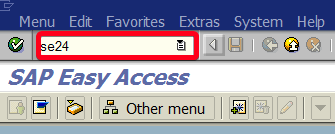Step 2. Choose class “ZCL_CALCULATOR“. If you don’t have this class yet you can follow this tutorial. Of course, a unit test can be created for any other customer classes?Step 3. Click on Change button: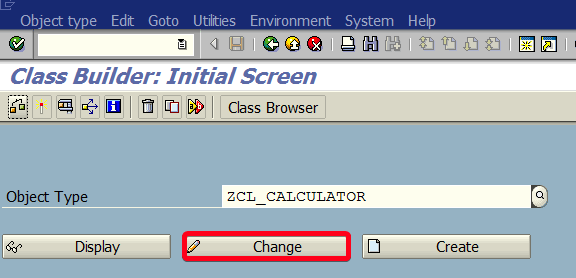Step 4. Click on Local Test Classes button: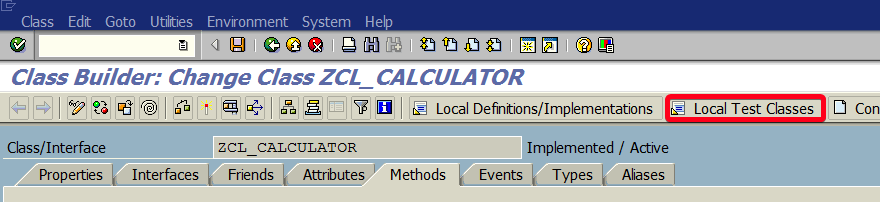Step 5. First, we will create a unit test for ADD method. You need to put following ABAP code in local test class source file:

[cc lang=”abap”]

CLASS abap_unit_testclass DEFINITION “#AU Duration Short
FOR TESTING. “#AU Risk_Level Harmless
PRIVATE SECTION.
ENDCLASS.

CLASS abap_unit_testclass IMPLEMENTATION.
DATA result TYPE int4.

result = zcl_calculator=>add( i_a = 5 i_b = 6 ).
cl_aunit_assert=>assert_equals(
act = result
exp = 11
).
ENDMETHOD.

ENDCLASS.

[/cc]

Step 6. It should look like this: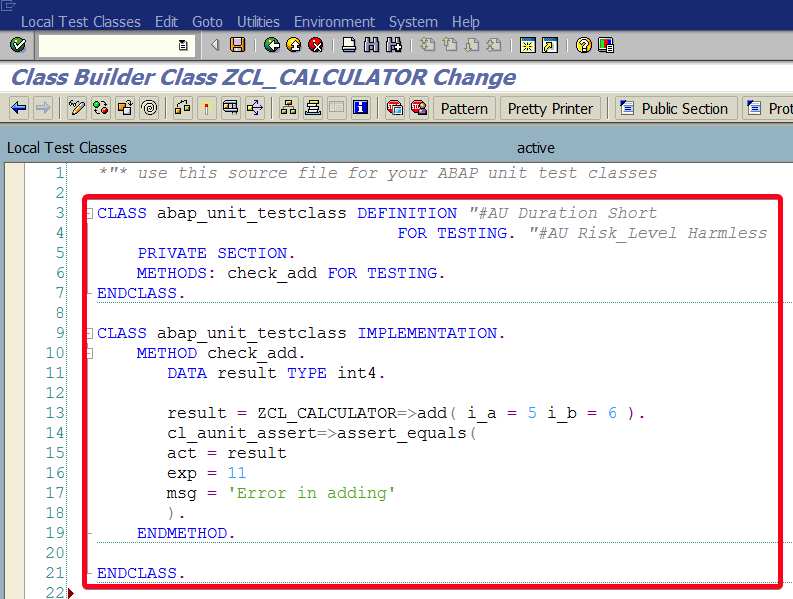Step 7. Add methods that will check other parts of ZCL_CALCULATOR class. The final code should look like this:

[cc lang=”abap”]

CLASS abap_unit_testclass DEFINITION “#AU Duration Short
FOR TESTING. “#AU Risk_Level Harmless
PRIVATE SECTION.
check_substract FOR TESTING,
check_multiplicy FOR TESTING,
check_divide FOR TESTING.
ENDCLASS.

CLASS abap_unit_testclass IMPLEMENTATION.
DATA result TYPE int4.

result = zcl_calculator=>add( i_a = 5 i_b = 6 ).
cl_aunit_assert=>assert_equals(
act = result
exp = 11
).
ENDMETHOD.

METHOD check_substract.
DATA result TYPE int4.

result = zcl_calculator=>substract( i_a = 7 i_b = 5 ).
cl_aunit_assert=>assert_equals(
act = result
exp = 2
msg = ‘Error in substraction’
).
ENDMETHOD.

METHOD check_multiplicy.
DATA result TYPE int4.

result = zcl_calculator=>multiply( i_a = 5 i_b = 6 ).
cl_aunit_assert=>assert_equals(
act = result
exp = 30
msg = ‘Error in multiplication’
).
ENDMETHOD.

METHOD check_divide.
DATA result TYPE int4.

result = zcl_calculator=>divide( i_a = 8 i_b = 2 ).
cl_aunit_assert=>assert_equals(
act = result
exp = 4
msg = ‘Error in dividing’
).
ENDMETHOD.
ENDCLASS.

[/cc]

Step 8. Active Unit Test class using Activate button:Step 9. Now you can use your unit test class to test ZCL_CALCULATOR. Choose Local Test Class | Execute | Unit Tests from the top menu:Step 10. If everything went well and all test are passed you should see status on the bottom of screen: